# Statistics

(diff) ← Older revision | Latest revision (diff) | Newer revision → (diff)

A term used in mathematical statistics as a name for functions of the results of observations.

Let a random variabletake values in the sample space. Any-measurable mappingfrom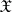onto a measurable spaceis then called a statistic, and the probability distribution of the statisticis defined by the formula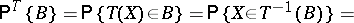### Examples.

1) Letbe independent identically-distributed random variables which have a variance. The statistics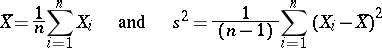are then unbiased estimators for the mathematical expectation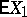and the variance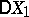, respectively.

2) The terms of the variational series (series of order statistics, cf. Order statistic)constructed from the observations, are statistics.

3) Let the random variablesform a stationary stochastic process with spectral density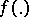. In this case the statisticcalled the periodogram, is an asymptotically-unbiased estimator for, given certain specific conditions of regularity on, i.e.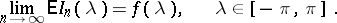In the theory of estimation and statistical hypotheses testing, great importance is attached to the concept of a sufficient statistic, which brings about a reduction of data without any loss of information on the (parametric) family of distributions under consideration.

How to Cite This Entry:
Statistics. Encyclopedia of Mathematics. URL: http://encyclopediaofmath.org/index.php?title=Statistics&oldid=14854
This article was adapted from an original article by M.S. Nikulin (originator), which appeared in Encyclopedia of Mathematics - ISBN 1402006098. See original article